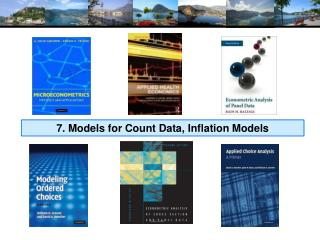DownloadDownload Presentation7. Models for Count Data, Inflation Models

# 7. Models for Count Data, Inflation Models

Download Presentation## 7. Models for Count Data, Inflation Models

- - - - - - - - - - - - - - - - - - - - - - - - - - - E N D - - - - - - - - - - - - - - - - - - - - - - - - - - -
##### Presentation Transcript

1. 7. Models for Count Data, Inflation Models

2. Models forCount Data

3. Doctor Visits

4. Basic Model for Counts of Events E.g., Visits to site, number of purchases, number of doctor visits Regression approach Quantitative outcome measured Discrete variable, model probabilities Nonnegative random variable Poisson probabilities – “loglinear model”

5. Efficiency and Robustness • Nonlinear Least Squares • Robust – uses only the conditional mean • Inefficient – does not use distribution information • Maximum Likelihood • Less robust – specific to loglinear model forms • Efficient – uses distributional information • Pseudo-ML • Same as Poisson • Robust to some kinds of nonPoissonness

6. Poisson Model for Doctor Visits

7. Alternative Covariance Matrices

8. Partial Effects

9. Poisson Model Specification Issues Equi-dispersion: Var[yi|xi] = E[yi|xi]. Overdispersion: If i = exp[’xi + εi], E[yi|xi] = γexp[’xi] Var[yi] > E[yi] (overdispersed) εi ~ log-Gamma  Negative binomial model εi ~ Normal[0,2]  Normal-mixture model εi is viewed as unobserved heterogeneity (“frailty”). Normal model may be more natural. Estimation is a bit more complicated.

10. Overdispersion • In the Poisson model, Var[y|x]=E[y|x] • Equidispersion is a strong assumption • Negbin II: Var[y|x]=E[y|x] + 2E[y|x]2 • How does overdispersion arise: • NonPoissonness • Omitted Heterogeneity

11. Negative Binomial Regression

12. NegBin Model for Doctor Visits

13. Poisson (log)Normal Mixture

14. Negative Binomial Specification Prob(Yi=j|xi) has greater mass to the right and left of the mean Conditional mean function is the same as the Poisson: E[yi|xi] = λi=Exp(’xi), so marginal effects have the same form. Variance is Var[yi|xi] = λi(1 + α λi), α is the overdispersion parameter; α = 0 reverts to the Poisson. Poisson is consistent when NegBin is appropriate. Therefore, this is a case for the ROBUST covariance matrix estimator. (Neglected heterogeneity that is uncorrelated with xi.)

15. Testing for Overdispersion Regression based test: Regress (y-mean)2 on mean: Slope should = 1.

16. Wald Test for Overdispersion

17. Partial Effects Should Be the Same

18. Model Formulations for Negative Binomial E[yi |xi ]=λi

19. NegBin-1 Model

20. NegBin-P Model NB-2 NB-1 Poisson

21. Censoring and Truncation in Count Models • Observations > 10 seem to come from a different process. What to do with them? • Censored Poisson: Treat any observation > 10 as 10. • Truncated Poisson: Examine the distribution only with observations less than or equal to 10. • Intensity equation in hurdle models • On site counts for recreation usage. Censoring and truncation both change the model. Adjust the distribution (log likelihood) to account for the censoring or truncation.

22. Effect of Specification on Partial Effects

23. Two Part Models

24. Zero Inflation?

25. Zero Inflation – ZIP Models Two regimes: (Recreation site visits) Zero (with probability 1). (Never visit site) Poisson with Pr(0) = exp[- ’xi]. (Number of visits, including zero visits this season.) Unconditional: Pr = P(regime 0) + P(regime 1)*Pr[0|regime 1] Pr[j | j >0] = P(regime 1)*Pr[j|regime 1] This is a “latent class model”

26. Zero Inflation Models

27. Notes on Zero Inflation Models • Poisson is not nested in ZIP. γ= 0 in ZIP does not produce Poisson; it produces ZIP with P(regime 0) = ½. • Standard tests are not appropriate • Use Vuong statistic. ZIP model almost always wins. • Zero Inflation models extend to NB models – ZINB(tau) and ZINB are standard models • Creates two sources of overdispersion • Generally difficult to estimate

28. An Unidentified ZINB Model

29. Partial Effects for Different Models

30. The Vuong Statistic for Nonnested Models

31. A Hurdle Model Two part model: Model 1: Probability model for more than zero occurrences Model 2: Model for number of occurrences given that the number is greater than zero. Applications common in health economics Usage of health care facilities Use of drugs, alcohol, etc.

32. Hurdle Model

33. Hurdle Model for Doctor Visits

34. Partial Effects